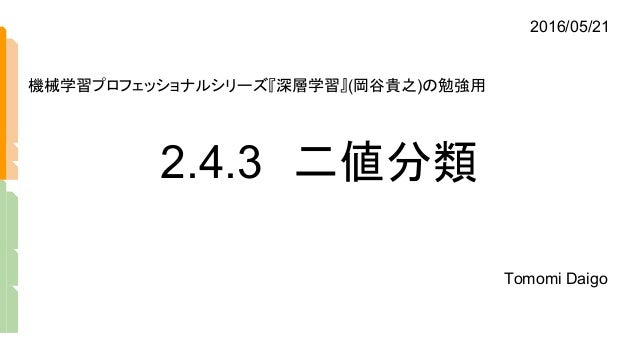Successfully reported this slideshow.
We use your LinkedIn profile and activity data to personalize ads and to show you more relevant ads. You can change your ad preferences anytime.Upcoming SlideShare
Loading in …5
×

# 2値分類・多クラス分類

『深層学習』（岡谷貴之）のChapter2.4.3および2.4.4を解説した。

• Full Name
Comment goes here.

Are you sure you want to Yes No
Your message goes here• Be the first to comment

### 2値分類・多クラス分類

1. 1. 2.4.3　二値分類 Tomomi Daigo 機械学習プロフェッショナルシリーズ『深層学習』(岡谷貴之)の勉強用 2016/05/21
2. 2. 二値分類の例 男 女 ？ ・ ・ ・ ・ ・ ・ 0.1 0.2 0.0 ・ ・ ・　 0.1 １ （y >= 0.5 ） y 0 （y < 0.5 ） 分布
3. 3. Xn y X1 X2 X3 X・・・ 1 0.5 0 2値分類におけるモデルの入出力のイメージ。 0.5を境にそれ以上は1、以下は0とする。 1 0 イメージ 分布
4. 4. 分類結果を確率で考える p(d=1|x) 「（d＝１）となる事後確率」＝入力xを受け取ったとき、その正解データdが１である確率 モデルがこの確率を出力することにしたい。 分布
5. 5. ・ ・ ・ ・ ・ ・ NNモデルの出力yは、 p（d=1|x）[あるデータｘを受け取った時、その正解データが1である確率] を表す yｘ y = 1 + e-u 1 出力のロジスティック関数の値域は [0,1]。 確率とみなす。 p(d=1|x) ≈ y(x；w) （p27上部） 分布
6. 6. 重さ（ｇ） 数（個） 0 分布 100 200 50 25 ex) 100個のイモの重さを調べる 分布
7. 7. モデルの分布イメージ 正解の分布と近づける Xn d X1 X2 X3 X・・・ 1 0 Xn y X1 X2 X3 X・・・ 1 0.5 0 1 0 正解 訓練モデル 間違い 分布
8. 8. 正解d=1 「事後分布」？ d=0の時、事後確率はp(d=0|x) y(x2)を1と判断する確率はこの図で は0.2。裏返すと、y(x2)を0と判断す る確率は、1-0.2=0.8 事後分布　p（d｜x；w） モデルのパラメータwのもとで、あるデータxが与えられた時、それが正解dである確率の分布 y X1 1 0.5 0 y X2 1 0.5 0 d=1の時、事後確率はp(d=1|x)。 y(x1)をd、つまり1と判断する確率 はこの図では0.7 0.7 正解d=0 0.2 分類を間違えた例。 y(x3)=0の確率が1-0.7=0.3 本来なら1に近づかせたい。 y X3 1 0.5 0 正解d=0 0.7 分布
9. 9. d=0の時、事後確率はp(d=0|x) y(x1)を1と判断する確率はこの図で は0.2。裏返すと、y(x1)を0と判断す る確率は、1-0.2=0.8 「確率の分布」って何？ 事後分布　p（d｜x；w） モデルのパラメータwのもとで、あるデータxが与えられた時、それが正解dである確率の分布 y X1 1 0.5 0 y X1 1 0.5 0 d=1の時、事後確率はp(d=1|x)。 y(x1)を1と判断する確率はこの図 では0.7 0.7 正解d=1 正解d=0 0.2 分類を間違えた例。 y(x1)=0の確率が1-0.7=0.3 本来なら1に近づかせたい。 y X1 1 0.5 0 正解d=0 0.7 X1 1 0.5 0 X2 X3 0.7 0.2 1-0.7=0.3 「事後分布」 0.7, 0.2, 0.3….がモデルのyの事後分布（事後確率の分布）？ ちなみに、正解の確率分布は、1,1,1,1,....になる。はず。 正解が正解である確率は常に1だから 正解がd=0の時、正 解である確率は 1-y(x)になることに注 意！ 分布
10. 10. p(d|x) = p(d = 1|x)d p(d = 0|x)1-d (2.7) あるデータxが与えられた時、それが正解dである確率（の分布） ＊）d=1ではない場合はd=0である。 ベルヌーイ分布f(k;p) = pk (1-p)1-k　 と一致している 式で書く 分布
11. 11. ベルヌーイ分布 成功 or 失敗 f(k;p) = pk (1-p)1-k　 ある確率Pで成功する事象が、成功（k=1）または失敗(k=0)する確率の分布。 1回の試行について考えるもの。N回のうちk回成功する確率をnCk pk (1-p)n-k　 とす る二項分布を導ける。 確率 成功 失敗 p 1-p 分布
12. 12. p(d|x) = p(d = 1|x)d　 p(d = 0|x)1-d (2.7) あるデータxが与えられた時、それが正解d（1または0）である確率 d=1に対応。 あるデータｘの正解が d=1のとき、式のこちら側を使う。 あるｘを受け取った時、それが正解 d=1である確率の1乗 d=0に対応。 あるデータｘの正解が d=0のとき、式のこちら側を使う。 あるｘを受け取った時、それが正解 d=0である確率の1-0乗 右辺の片側を使った時、もう片側は xxx の0乗となり、常に1である 右肩のdのイメージは「スイッチ」 分布
13. 13. p(d|x) = p(d = 1|x)d　 p(d = 0|x)1-d (2.7) あるデータxが与えられた時、それが正解d（1または0）である確率 d=1に対応。 あるデータｘの正解が d=1のとき、式のこちら側を使う。 あるｘを受け取った時、それが正解 d=1である確率の1乗 d=0に対応。 あるデータｘの正解が d=0のとき、式のこちら側を使う。 あるｘを受け取った時、それが正解 d=0である確率の1-0乗 右辺の片側を使った時、もう片側は xxx の0乗となり、常に1である この表記方法は、d=1,0両方の場合を考えた事後分布を一つの式で表せる 分布
14. 14. N個のデータ全部を正解させたい ・ ・ ・ ・ ・ ・ y = dn ｘn W 全部正解になるようなモデルのパラメー タWを求めたい！一番尤もらしい Wを。 すべての訓練データ{(xn,dn)|n=1,...,N}について、モデルにxnを入力するとき、出力y はその都度dnと一致してほしい。（つまり、全部正解であってほしい。） 正解を出力してくれるWの、尤もらしさ「尤度」を求める 尤度
15. 15. L(w) = Πp(dn |xn ;w) = Π{y(xn ;w)}dn {1 - y(xn ;w)} 1-dn p(d|x) = p(d = 1|x)d p(d = 0|x)1-d (2.7) モデルの出力y=[xを受け取ったときのd＝1の確率]なので、2.7式と対応。 違いは、訓練データxnに対して、全データ分(n個)の出力を掛けあわせること。 p(d|x)は正解において1をとり、その積であるL(w)は1が最大。 L(w)=1であるとき、すべてのXnについてyが正解を出力したことになる。 n=1 N n=1 N 尤度
16. 16. L(w) = Πp(dn |xn ;w) = Π{y(xn ;w)}dn {1 - y(xn ;w)} 1-dn 今、x1が与えられて、正解はd=1だったとする。 モデルは良く出来ていて（wがよい値で）yが0.9を出力した。 この時、d1=1であるため、xxx の項のみがn=1でのL(w)に寄与するが、 yは0.9を出力しているので、n=1でのL(w)は0.9と、非常に尤もらしい。 逆に、d=1であるにもかかわらず、yが0.1を出力したとする（d=0と判断してしまった）。 この場合は、xxx の項を使用するが、yの値は0.1。L(w)全体の掛け合わせの中で、0.1と いう小さい値を掛けあわせることになり、その結果L(w)の値は減少する。 もしも、d=0だったなら、xxx の項がアクティブになり、(1-0.1)=0.9という大きな値が結果に 寄与できたのに・・・。 同様に、すべてのnについて、yがdに近い値を出力すれば、L(w)は1に近づき、wは非常 に尤もらしい。 n=1 N n=1 N （説明） 尤度
17. 17. （　） 尤度？ あるパラメタを指定した時、あるデータが再現できる確率 （http://www.slideshare.net/logics-of-blue/2-2-25620649） ex) パラメタ：コインが表になる確率 データ：1回表、1回裏 1 3 1 3 × 1 -（　 ） 2 9 = 1 3 パラメタが のとき 1 2 1 2 × 1 - 1 4 = 1 2 パラメタが のとき 尤度 尤度
18. 18. 最小化問題にしたい E(w) = - ∑ [dn log y(xn ;w) + (1 - dn ) log {1 - y(xn ;w)}] (2.8) n=1 N 先ほどの尤度L(w)の式の対数を取れば導出できる。この式を誤差関数と呼ぶ。 値域は、0<=E(w)？最小化は0を目指すことになる 尤度
19. 19. E(w) = - ∑ [dn log y(xn ;w) + (1 - dn ) log {1 - y(xn ;w)}] (2.8) n=1 N yはモデルの出力なので確率（0~1） L(w) = Πp(dn |xn ;w) = Π{y(xn ;w)}dn {1 - y(xn ;w)} 1-dn ①　log（a×b）=log a + log b ②　log■x = x log■　　　 の規則で変形可能　 ※このマイナスは式変形では導けない。 最小化のために付け加えたもの ｌog yはe(=2.718…)をyにするために必要なべき乗数なので-∞ ～0 (eの-∞乗≒0(近似的に), eの0乗=1） 本当はeを何乗しても0になりませんし、log yの値は小さくてもせいぜい-■▲(2桁)程度だと思います。
20. 20. E(w) = - ∑ [dn log y(xn ;w) + (1 - dn ) log {1 - y(xn ;w)}] (2.8) n=1 N dnは0または１なので、やはり下線部は（負の数）～0 L(w) = Πp(dn |xn ;w) = Π{y(xn ;w)}dn {1 - y(xn ;w)} 1-dn 元の尤度の式は確率yの積なので0~1しか取らない。 最大化した場合１となる 右側ブロックも同じ計算で、その和∑も（負の数）～0。和はデータ数n分あるので、普通に絶対値の大きい値になる 式全体は頭に-が付いているのでマイナスをかけて（正の値）～０　つまり　０～（正の値）となり、 この式で言う最小化は０を目指すことになる。誤差関数E(w)＝０→「誤差がない」
21. 21. 「モデルの出力＝確率」の証明 p(x,d=1) p(x,d=0)+p(x,d=1) 条件付き確率の定義　p(d=1|x) = p(d=1∧x)/p(x)　より。 p(x)は、xはd=0のものとd=1のものからなるので、その和で書ける。 xxxの意味は、[存在するすべてのxの確率のうち、d=1であるxの割合]。 p(d=1|x) = p17下部 p(d=1|x) ≈ y(x；w) （p27上部）　 より、モデルの出力yはxをもらってd=1である確率としていた。 証明
22. 22. 1 + e-u 1 ところで、モデルの出力yにはロジスティック関数をかませていたので・・・ p(x,d=1) p(x,d=0)+p(x,d=1) =p(d=1|x) = p(x,d=1) p(x,d=0) u ≡ log とおけば、 のはず。 変形して、 u= log p(x,d=1) - log p(x,d=0)をロジスティック関数に代入すると、 exp(loge X)=Xより、xxxとzzzは一致する。 ゆえに、出力をロジスティック関数にすれば、モデルの出力y(x;w)= p(d=1|x) (p17) の 前提は妥当であった。 = y(x；w) 証明
23. 23. 2.4.4　多クラス分類
24. 24. どのクラスっぽいか？ ・ ・ ・ ・ ・ ・ y1 y2 yk LL-1 w11 w12 yk = zk = ∑ euj euk 例えばMNISTの画像分類のような問題。 k個の 出力yへの入力ukにつき、それぞれソフトマック ス関数を適用する。ソフトマックス関数の値域 も[0,1]。こちらも確率とみなす。 ｊ＝１ K （L） （L） u1 u2 uk k個の出力yの総和（＝１）のうち、その出力 kが 占める割合。 ｚ1 ｚ２ ｚ3 モデル
25. 25. ・ ・ ・ ・ ・ ・ y1 y2 yk LL-1 w11 w12 p(Ck|x) = yk = zk = ∑ euj euk ｊ＝１ K （L） （L） u1 u2 uk 2値分類同様に考えて、ある xが与えられて、そ れがクラスCkである確率をp(Ck|x)とすると、 0.1 0.7 0.2 モデルの出力ykは、xがそのykの対応クラスCkに属する 確率となる。←の例では p(C1)=0.1, p(C2)=0.7, p(C3)=0.2 このクラスっぽい！ モデル
26. 26. ・ ・ ・ ・ ・ ・ y1 y2 yk LL-1 w11 w12u1 u2 uk また、訓練データの正解 dは、 0.1 0.7 0.2 0 1 0 = dn = [0 1 0]T のように表現する。「ワンホットベクトル」とも呼ぶ。 対応 「ワン・ホット・エンコーディング」という表現法 モデル
27. 27. モデルの出力＝分布 [xを受け取って、そのxがあるクラスである確率]（分布）を、一般化する。 p(d|x) = Π p(Ck|x)dk K k=1 分布 p(Ck |x) = yk = zk (L) モデルの出力を、[xを受け取って、そのxがあるクラスである確率]（分布）とする。
28. 28. この式も右肩のdがスイッチになって、 p(d|x) = Π p(Ck|x)dk K k=1 = p(C1|x)d1 p(C2|x)d2 p(C3|x)d3 ・・・p(Ck|x)dk クラスC1である確率 クラスC2である確率 クラスC3である確率 クラスCkである確率 各クラス、自分のクラスの順番じゃない時は1になって寄与しないので・・・ → p(C1|x)or p(C2|x) or p(C3|x) or ・・・ or p(Ck|x) のどれかを計算に使う。 dk = [0 1 0...]T であることに注意。 分布
29. 29. 同様に、モデルの出力を考えて、 L(w)=Πp(dn|xn;w) = Π Π p(Ck|x)dnk = Π Π (yk(xn;w))dnk K k=1 = y1(x1;w)d11 y2(x1;w)d12 y3(x1;w)d13 ・・・yk(x1;w)d1k クラスC1である確率 クラスC2である確率 クラスC3である確率 クラスCkである確率 K k=1 N n=1 K k=1 N n=1 × y1(x2;w)d21 y2(x2;w)d22 y3(x2;w)d23 ・・・yk(x2;w)d2k × y1(x3;w)d31 y2(x3;w)d32 y3(x3;w)d33 ・・・yk(x3;w)d3k ・・・× y1(xn;w)dn1 y2(xn;w)dn2 y3(xn;w)dn3 ・・・yk(xn;w)dnk n=1のブロック n=2のブロック n=3のブロック n=nのブロック 尤度
30. 30. 同様に、モデルの出力を考えて、 L(w)=Πp(dn|xn;w) = Π Π p(Ck|x)dnk = Π Π (yk(xn;w))dnk K k=1 = y1(x1;w)d11 y2(x1;w)d12 y3(x1;w)d13 ・・・yk(x1;w)d1k クラスC1である確率 クラスC2である確率 クラスC3である確率 クラスCkである確率 K k=1 N n=1 K k=1 N n=1 × y1(x2;w)d21 y2(x2;w)d22 y3(x2;w)d23 ・・・yk(x2;w)d2k × y1(x3;w)d31 y2(x3;w)d32 y3(x3;w)d33 ・・・yk(x3;w)d3k ・・・× y1(xn;w)dn1 y2(xn;w)dn2 y3(xn;w)dn3 ・・・yk(xn;w)dnk n=1のブロック n=2のブロック n=3のブロック n=nのブロック 2値分類同様、正解であるdk(dk=1となっているk)に対応する項のみ寄与し、 また、モデルの出力が不正解（dkの値から離れている）場合には小さな値として寄与して しまう。 結果、不正解のxnが多いほど、L(w)は小さな値を取る。（0に近づく） 正解が多いほど、1に近づく。 尤度が1に近づくようなwを求めれば、モデルは優れた分類ができるようになる。優れた分 類をするモデルのwを求めるために、尤度を使う。最大（極大）値になるwは微分で求めら れる(尤度をwで微分して結果が0になるようなwを求める)。 尤度
31. 31. 最小化する E(w) = - ∑ ∑ dnk log yk (xn ;w) （2.7） 2値分類同様、logをとってマイナスをつける。 KN k=1n=1 交差エントロピーと呼ばれる関数となる。 2値分類同様に、値域が0<=E(w)。最小値は0 尤度